Velocity-time (v-t) graph for a moving object is shown in the figure. Total displacement of the object during the time interval when there is non-zero acceleration and retardation is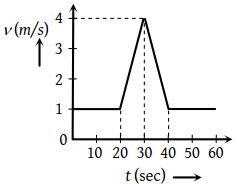(1) 60 m

(2) 50 m

(3) 30 m

(4) 40 m

Concept Questions :-

Graphs
High Yielding Test Series + Question Bank - NEET 2020

Difficulty Level:

For the velocity-time graph shown in figure below the distance covered by the body in last two seconds of its motion is what fraction of the total distance covered by it in all the seven seconds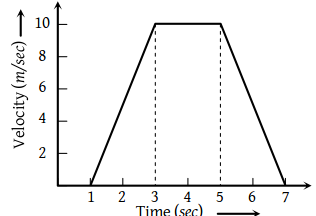(1) $\frac{1}{2}$

(2) $\frac{1}{4}$

(3) $\frac{1}{3}$

(4) $\frac{2}{3}$

Concept Questions :-

Graphs
High Yielding Test Series + Question Bank - NEET 2020

Difficulty Level:

In the following graph, distance travelled by the body in metres is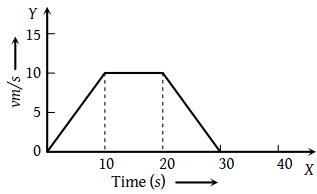(1) 200

(2) 250

(3) 300

(4) 400

Concept Questions :-

Graphs
High Yielding Test Series + Question Bank - NEET 2020

Difficulty Level:

Velocity-time curve for a body projected vertically upwards is

1. Parabola

2. Ellipse

3. Hyperbola

4. Straight line

Concept Questions :-

Graphs
High Yielding Test Series + Question Bank - NEET 2020

Difficulty Level:

The displacement-time graph of moving particle is shown below The instantaneous velocity of the particle is negative at the point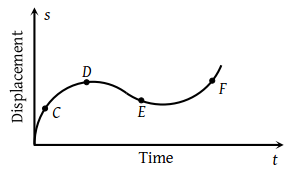(1) D

(2) F

(3) C

(4) E

Concept Questions :-

Instantaneous speed and instantaneous velocity
High Yielding Test Series + Question Bank - NEET 2020

Difficulty Level:

An object is moving with a uniform acceleration which is parallel to its instantaneous direction of motion. The displacement (s) – velocity (v) graph of this object is

 1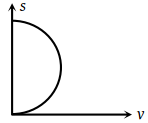2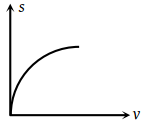3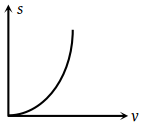4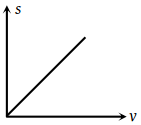Concept Questions :-

Uniformly accelerated motion
High Yielding Test Series + Question Bank - NEET 2020

Difficulty Level:

Which of the following graph represents uniform motion ?

 (1)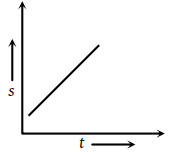(2)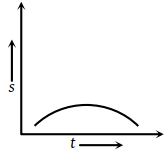(3)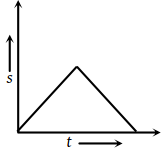(4)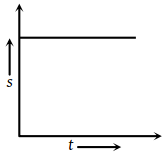Concept Questions :-

Average speed and average velocity
High Yielding Test Series + Question Bank - NEET 2020

Difficulty Level:

A ball is dropped vertically from a height d above the ground. It hits the ground and bounces up vertically to a height d/2. Neglecting subsequent motion and air resistance, its velocity v varies with the height h above the ground is

 (1)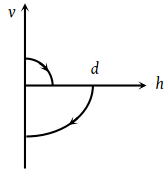(2)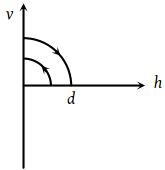(3)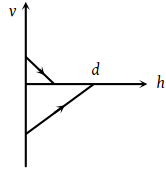(4)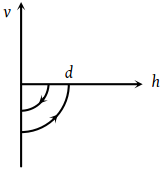Concept Questions :-

Graphs
High Yielding Test Series + Question Bank - NEET 2020

Difficulty Level:

The graph of displacement v/s time is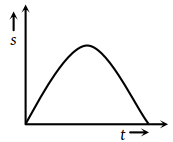Its corresponding velocity-time graph will be

 (1)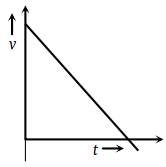(2)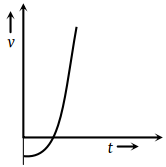(3)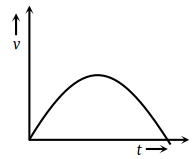(4)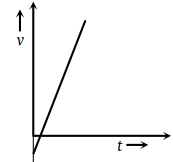Concept Questions :-

Graphs
High Yielding Test Series + Question Bank - NEET 2020

Difficulty Level:

A train moves from one station to another in 2 hours time. Its speed-time graph during this motion is shown in the figure. The maximum acceleration during the journey is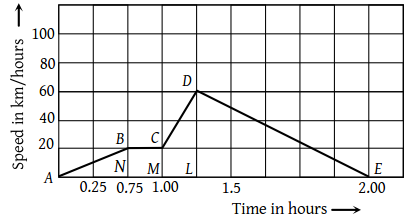(1) 140 km h–2

(2) 160 km h–2

(3) 100 km h–2

(4) 120 km h–2

Concept Questions :-

Graphs